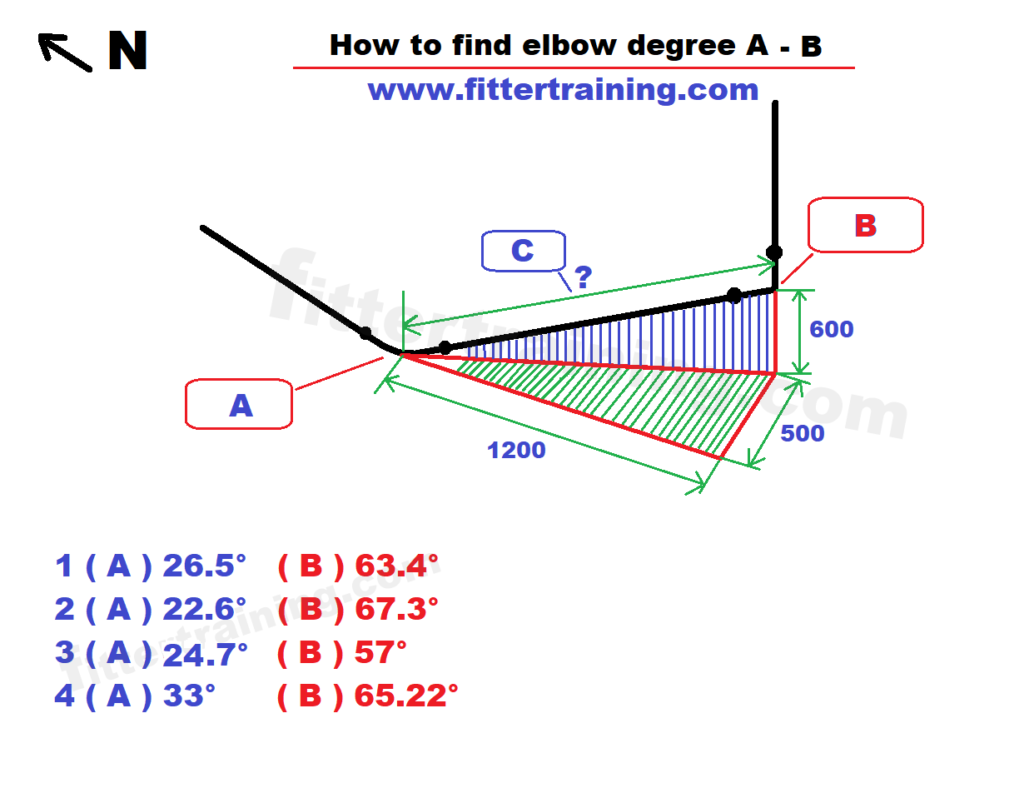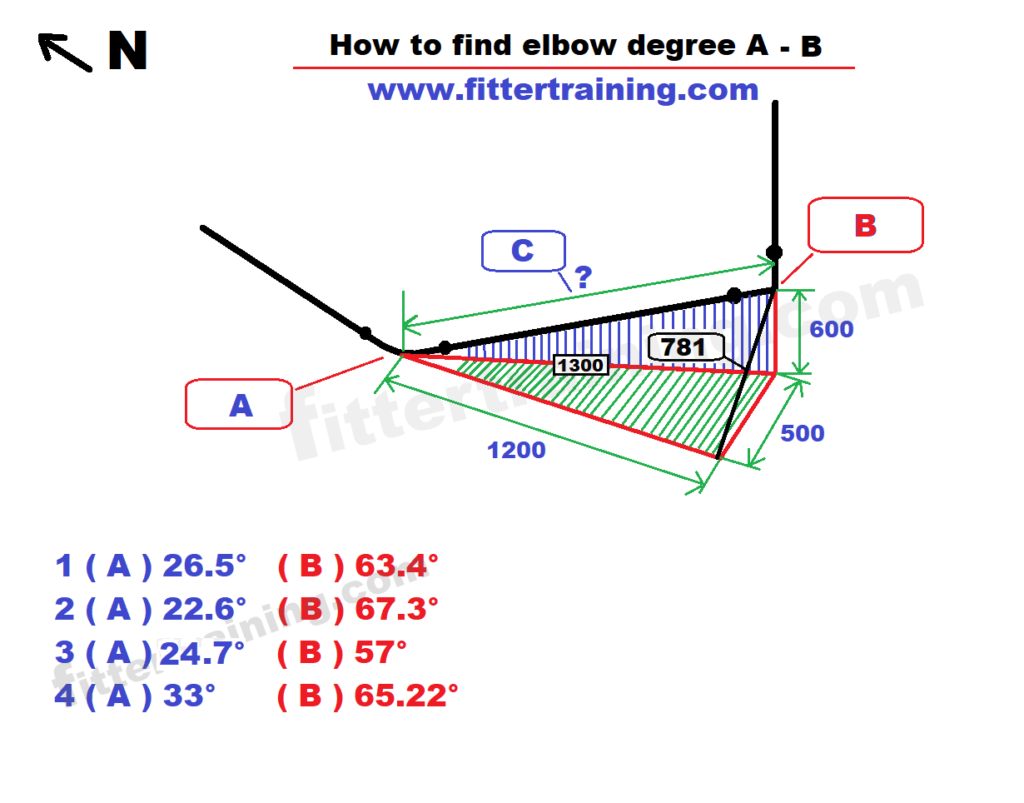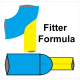Latest:

### Fitter training

piping and structural fitter fabricator training and pdf chart

# Double rolling pipe elbow degree and travel pipe length calculation

Double rolling pipe elbow degree calculation

Double rolling travel pipe length calculation

How to find elbow degree A & B

How to find  C dimensions

Pipe fitter and fabricator interview questions

Horizontal and vertical rolling pipe degree calculation1 (A ) 26.5°  ( B ) 63.4°

2 (A ) 22.6°  ( B ) 67.3°

3 (A ) 24.7°     ( B ) 57°

4 (A ) 33°     ( B ) 65.22°A elbow degree calculation

Tan-¹( 600 ÷ 1200 ) = 26.5°  wrong answer

Tan-¹( 500 ÷ 1200 ) = 22.6° wrong answer

Tan-¹( 600 ÷ 1300 ) = 24.7° wrong answer

√(500^² + 600^² = 781

Tan-¹(781 ÷ 1200 = 33° Right answer

B elbow degree calculation

Tan-¹( 1200 ÷ 600 ) = 63.4°  wrong answer

Tan-¹( 1200 ÷ 500 ) = 67.3°  wrong answer

90° – 33° = 57°   wrong answer

√(500^² + 1200^² = 1300

Tan-¹( 1300 ÷ 600 = 65.2° Right answer

C travel pipe length calculation

√( 1300^2 + 600^2 = 1431.7 mm

√(1200^2 + 781^2 = 1431.7 mm#### fitter training

Pipe fitter training, steel fitter training, piping and structural fitter fabricator training how to read piping and structural draining and Piping and structural pdf chart free download Lateral pipe tee branch Pipe fitter formula

### One thought on “Double rolling pipe elbow degree and travel pipe length calculation ”

•Amin Shafiei

Hi